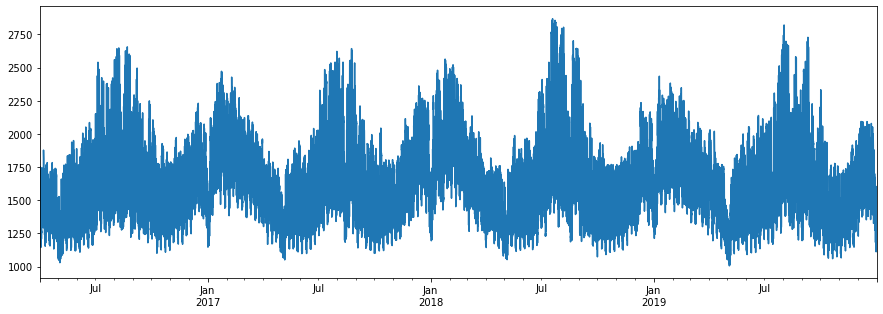# Introduction

I was holding a seminar on electricity usage forecast, and I heard that it is difficult to obtain it because the format of the past electricity consumption published by each electric power company is different. Therefore, I will summarize the data acquisition method for each electric power company.

By the way, the target power companies are Hokkaido Electric Power, Tohoku Electric Power, Tokyo Electric Power, Hokuriku Electric Power, Chubu Electric Power, Kansai Electric Power, Chugoku Electric Power, Shikoku Electric Power, Kyushu Electric Power, Okinawa Electric Power, and this time I will deal with Kansai Electric Power. ..

# Operating environment

It works in the environment called Colaboratory of Google.

Colaboratory

# Website

It seems that you can download the data from the following website.

Kansai Transmission and Distribution Power Supply and Demand Notice

#### `python`

``````
for y in range(2016, 2020):
for m in range(1,13):
url = "https://www.kansai-td.co.jp/yamasou/{:04}{:02}_jisseki.zip".format(y, m)
print(url)
!wget \$url

from glob import glob

files = glob("*.zip")
files.sort()

for f in files:
!unzip \$f
``````

#### `python`

``````
from glob import glob
import pandas as pd

files = glob("2*.csv")
files.sort()

df_juyo = pd.DataFrame()

for f in files:
print("\r", f, end="")
try:
df = pd.read_csv(f, encoding="Shift_JIS", skiprows=10, nrows=24)
d = df.DATE + " " + df.TIME
except:
df = pd.read_csv(f, encoding="Shift_JIS", skiprows=16, nrows=24)
#df.tail(25)
df_juyo = pd.concat([df_juyo, df])

print(df_juyo.shape)
print(df_juyo.columns)

df_juyo.index = pd.to_datetime(df_juyo["DATE"] + " " + df_juyo["TIME"])
df_juyo = df_juyo.sort_index()

df_juyo["Results on the day(10,000 kW)"].plot(figsize=(15,5))
``````did it!

Due to the large population of the area, it is inevitably a region that uses a lot of electricity.

Looking at the amount of electricity used, there are many things you notice. That's all for Kimura from the scene.

# Supplement

The person who read the article asked me, "It takes too much time, so what should I do if I want the data quickly?", So I decided to sell the data for a while. If you are interested in the data, please see the URL below.

https://ticket.tsuku2.jp/eventsDetail.php?ecd=16260900020422